To design models in SAP HANA, you'll require a thorough understanding of the essential keywords used most often during design time, such as the following:

• Measures and attributes
• Dimensions
• Fact tables
• Star Schemas
• Hierarchies
• Semantics
• Joins

In this blog post , we'll discuss these keywords in detail so you can understand the significance of using them to develop information views.

## Measures and Attributes

A data model is categorized into columns that are considered attributes and columns that are considered measures during design time to report the data accurately.

An attribute is an element you can use to define a relationship with a measure to fulfill your reporting needs. For example, an airline code, a flight number, or an aircraft type can all be considered measures.

Typically, attributes are essential so that you can define, in the model, the relevant relationships between attributes and measures, thus enabling end users to understand how calculations are performed and filtered to fulfill a particular reporting need. You might, for example, craft the model to answer the following questions:

• What is the total booking for a particular airline and airline type?
• What is the maximum capacity of the economy class by aircraft type?

A measure is any numeric column on which arithmetic operations (like SUM, AVERAGE, or TOP N values) can be performed. For example, to find the total bookings or the maximum capacity in economy class, etc. on which arithmetic expressions (such as SUM, AVERAGE, or TOP N values) and formulas can be used.

## Dimensions

Data models can include several attributes to be used to filter or aggregate measures. The reporting analysis can further be simplified by grouping these attributes into dimensions. In other words, a dimension can be used to group several attributes, as shown in the table below.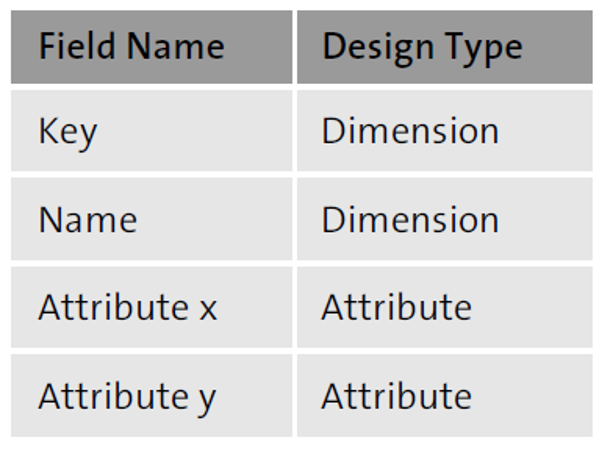Let's understand this distinction by considering the data sources SFLIGHT and SBOOK used by flight booking application.

In table SFLIGHT, the airline code can group attributes such as flight connection number and aircraft type, as shown here.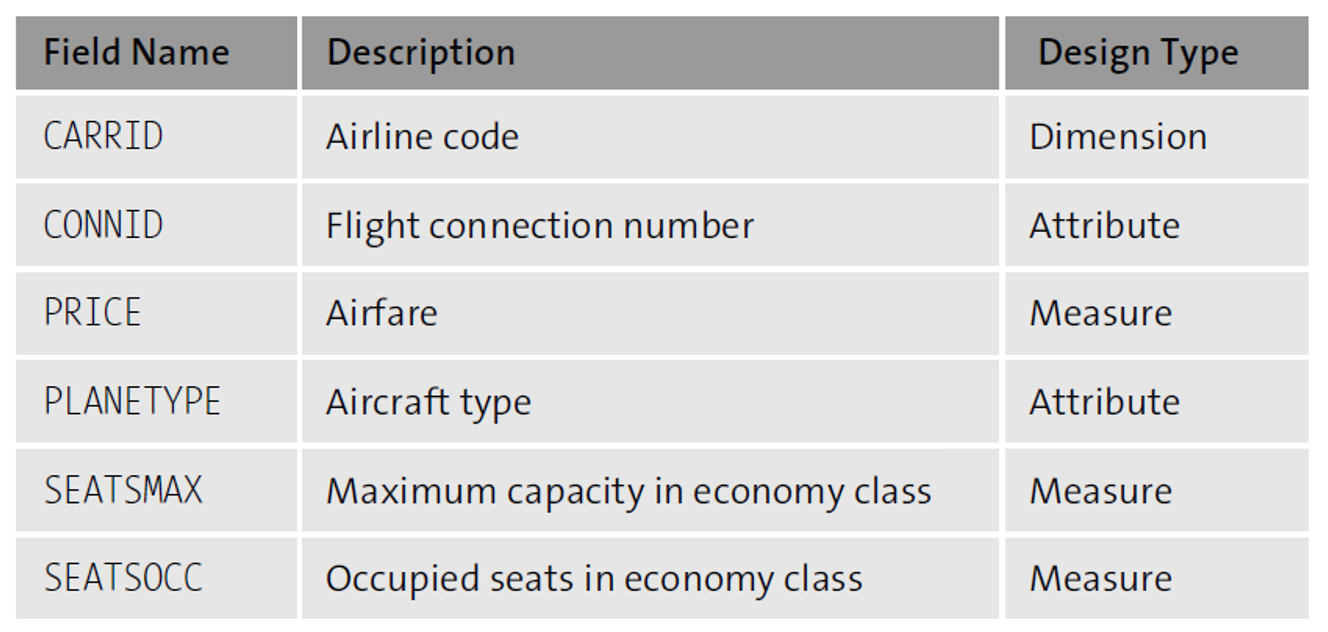Whereas in table SBOOK, the customer type can be used as a dimension to group attributes such as flight connection number and smoker, as shown here.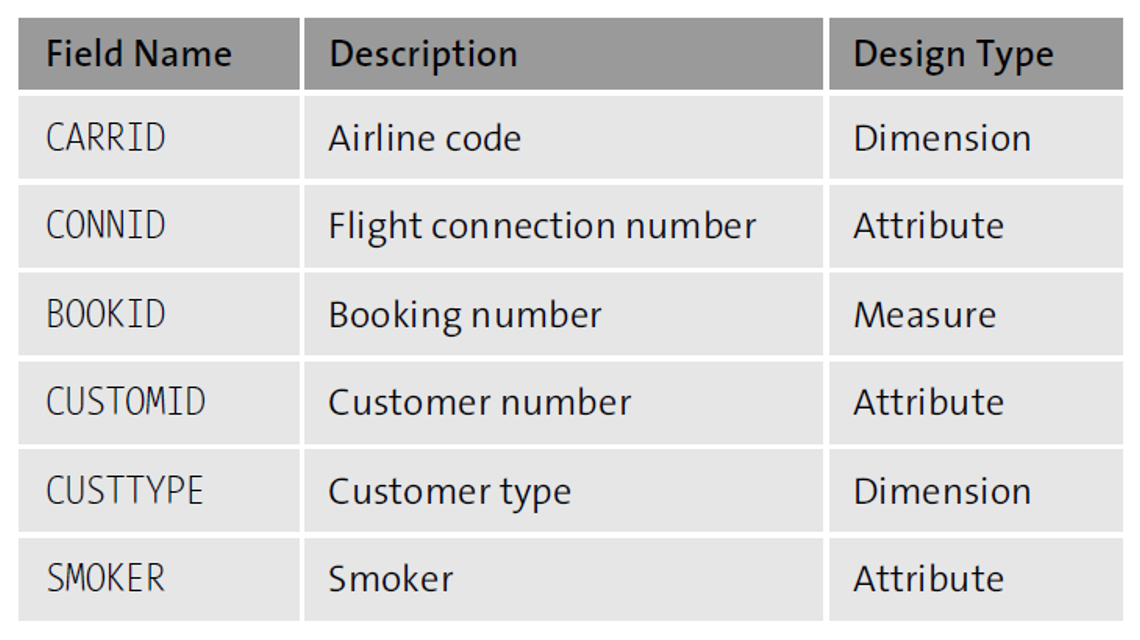## Fact Tables

A fact table consists of facts, measures, and keys used to identify each dimension's attributes—each dimension corresponding to a fact, as shown in the tables below.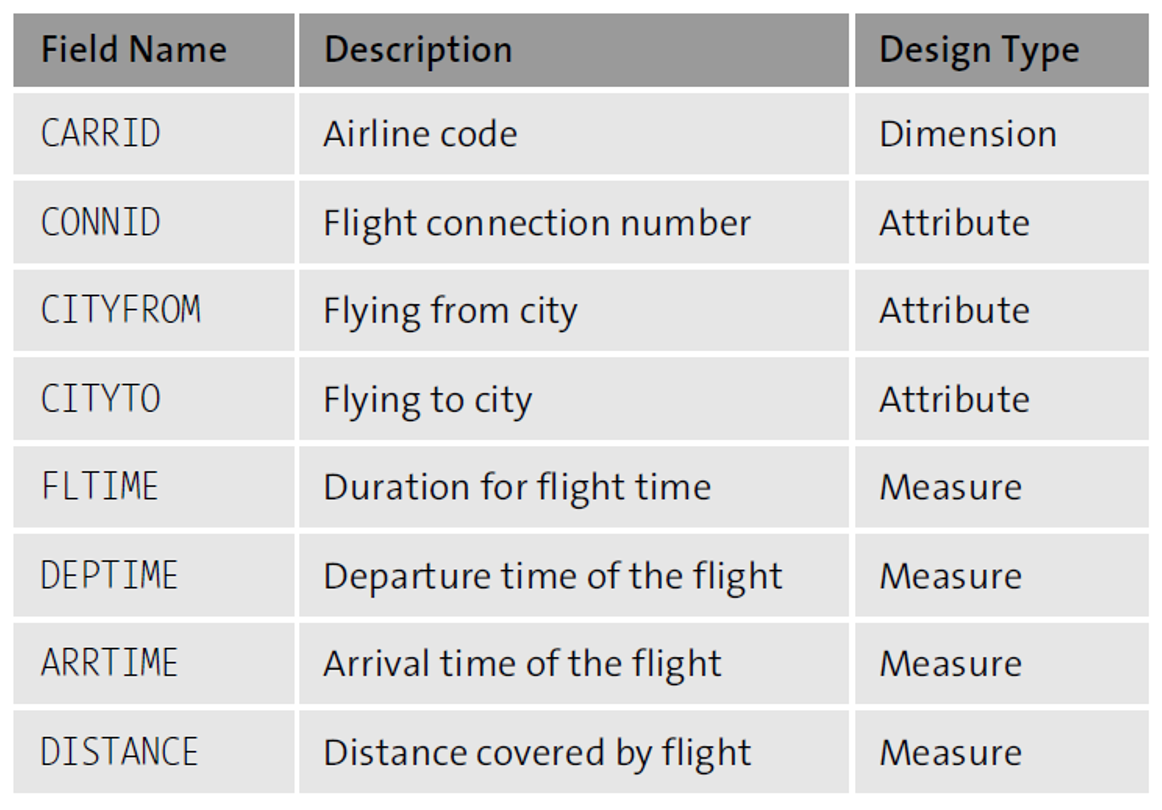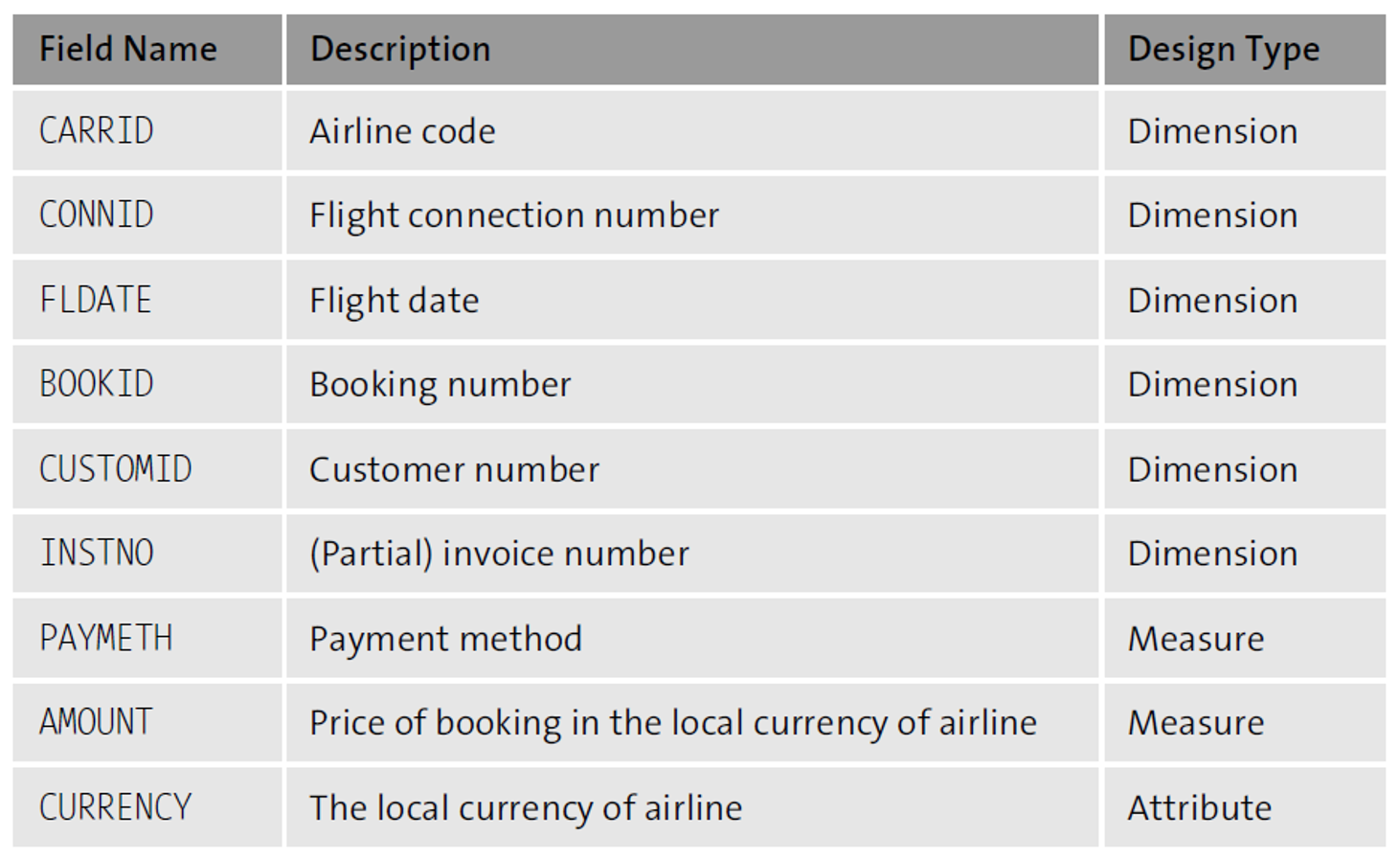## Star Schemas

As shown in the figure below, a star schema is defined when one fact table establishes a relationship with one or more dimension tables.

7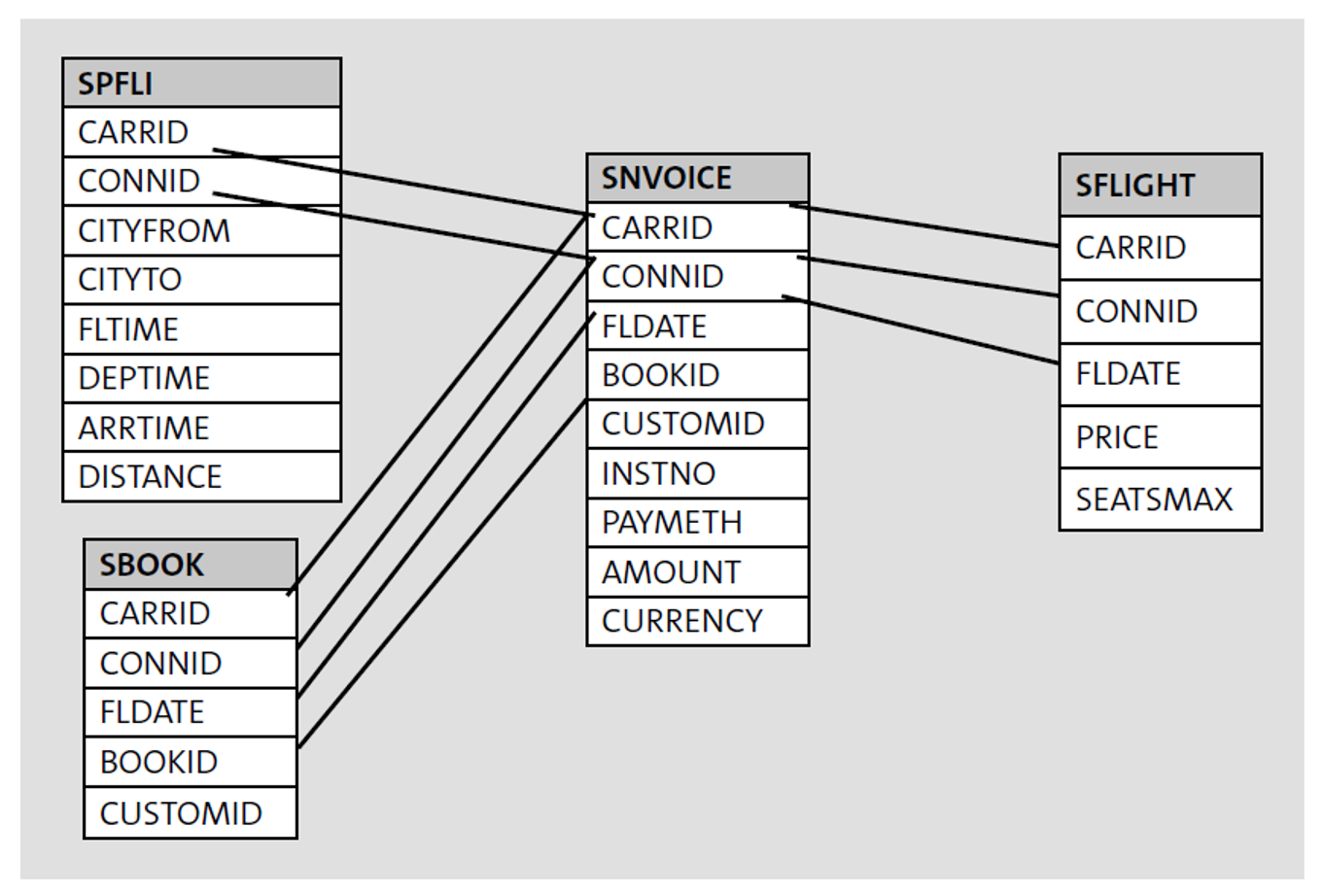## Hierarchies

A hierarchy represents the organization and offers drilldown capabilities to access the entire underlying set of information more easily while analyzing your data.

As shown in the following figure, for example, the hierarchy concept can be described as a group organized into several regions: North America, Europe, the Middle East, and Africa. Further, these regions can be split into corresponding countries, such as the United States, Canada, the United Kingdom, Germany, the United Arab Emirates (UAE), and Egypt.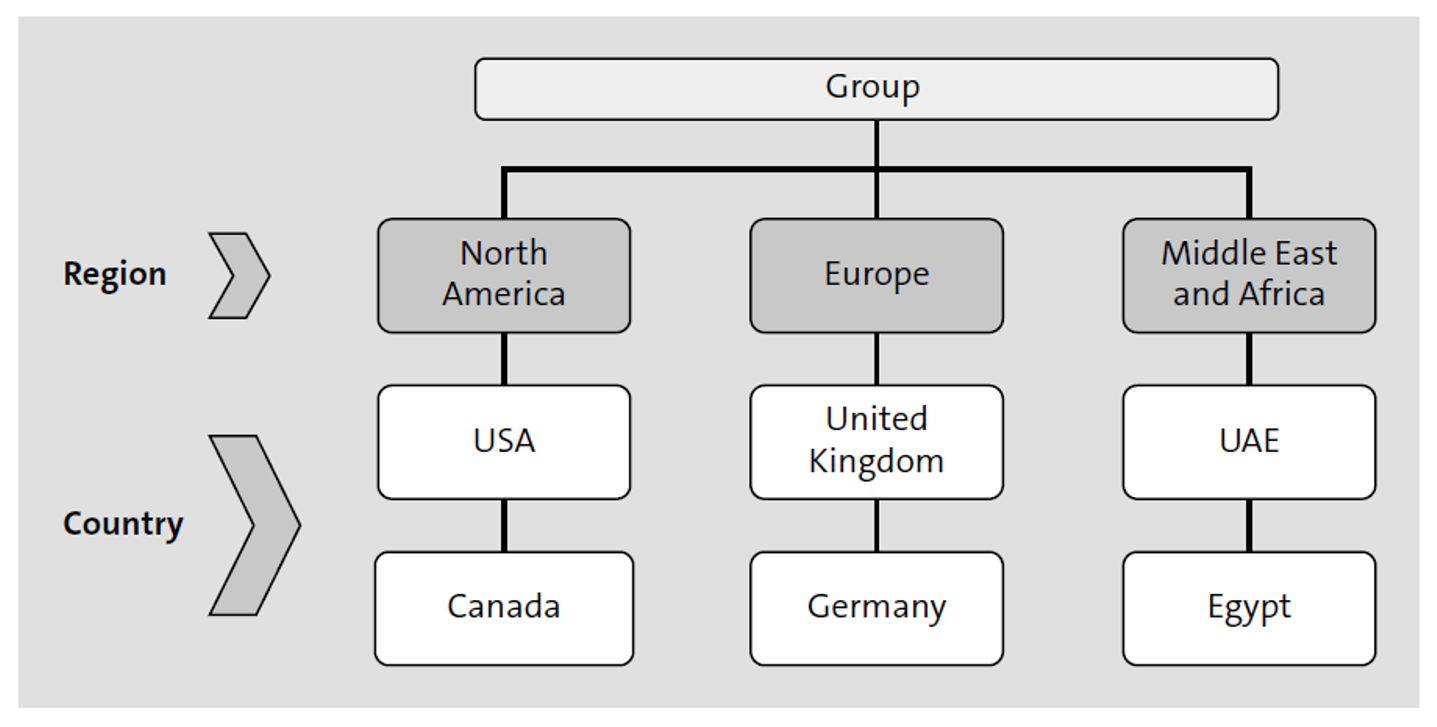## Semantics

The term semantics describes the categorization of the data used for reporting. The data reported can be of different types, such as monetary values, numbers of items, or percentages.

## Joins

Understanding the different join types SAP HANA supports is essential for relating source tables with each other to define optimal information models.

In real business scenarios, the data required to define information models will be distributed across several source tables, and to obtain the expected results, you may need to join these tables. This data, although spread across several tables, can be combined into one result set by using joins.

Several types of joins are possible in SAP HANA to obtain the desired results, such as the following:

• Inner joins
• Left outer joins
• Right outer joins
• Referential joins
• Text joins
• Temporal joins
• Star joins

### Inner Joins

An inner join is the most basic type of join used to retrieve data from two or more data sources. The join result set returns rows when at least one match meets the join conditions. The listing below shows an example of an inner join, in this case, with a join condition (CARRID) between the two tables SFLIGHT and SCARR.

`SELECT a~carrid, a~connid, b~carrname`

`   INTO TABLE @DATA(gt_results)`

`FROM sflight AS a INNER JOIN`

`    scarr AS b ON a~carrid = b~carrid`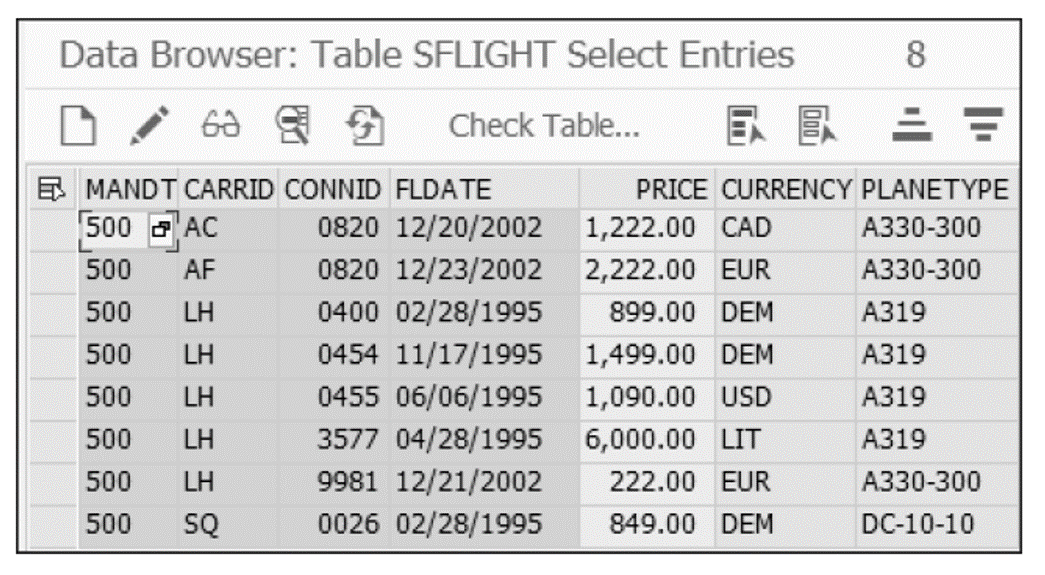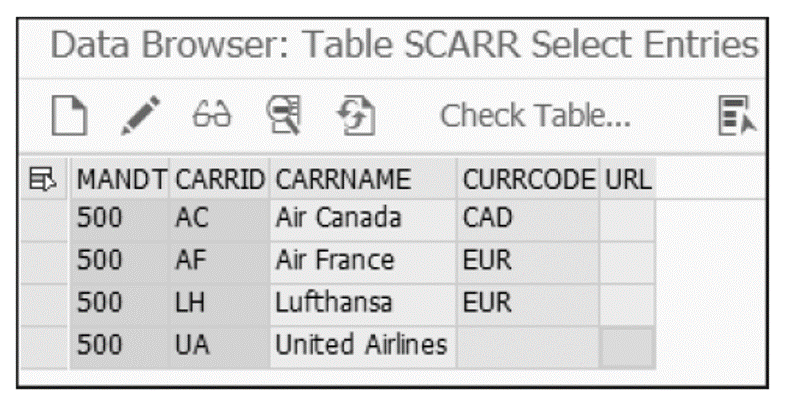The result set in the next figure shows all the rows of the SFLIGHT table that also have entries in table SCARR. Note that a row entry in table SFLIGHT (SQ as CARRID) has not been selected since that entry does not have a corresponding in table SCARR.

7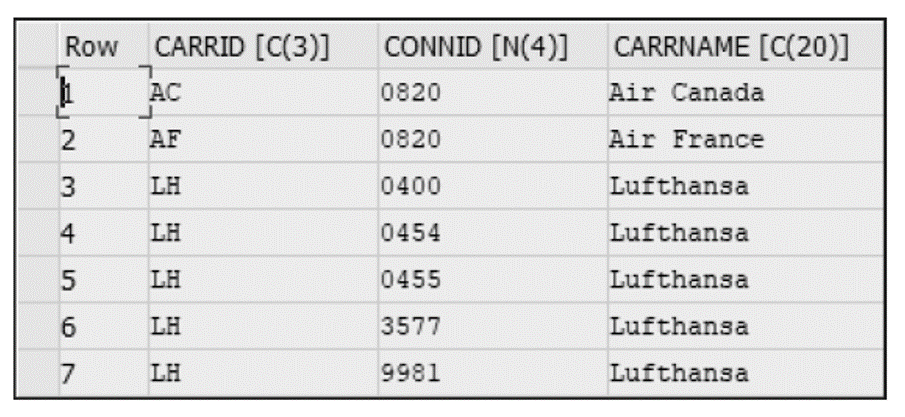### Left Outer Joins

With a left outer join, the result set returns all the rows from the left table even if no corresponding entry matches in the table on the right. The listing below shows an example of a left outer join with the tables SFLIGHT and SCARR (the same tables from the previous example), using the join condition CARRID.

`SELECT a~carrid, a~connid, b~carrname`

`   INTO TABLE @DATA(gt_left_outer_results)`

`FROM sflight AS a LEFT OUTER JOIN`

`    scarr AS b ON a~carrid = b~carrid`

Note that the entry in table SFLIGHT (with SQ as CORRID) is selected, but the corresponding entry in table SCARR is left blank in the result set (see figure below).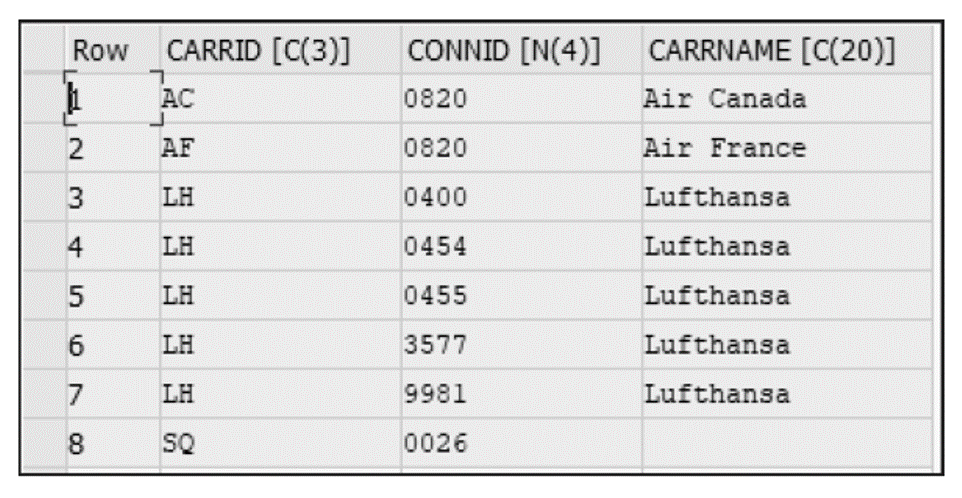### Right Outer Joins

With a right outer join, the result set returns all the rows from the table on the right even if no corresponding entry matches in the table on the left. Rarely used, this kind of join is mainly used in analytical views.

The number of records in the result set is determined by the number of records in the table on the right. Thus, the number of records in the result set can be greater than (but not less than) the number of records in the table on the left. The next listing shows an example of a right outer join between the tables SFLIGHT and SCARR.

`SELECT a~carrid, a~connid, b~carrname`

`   INTO TABLE @DATA(gt_right_outer_results)`

`FROM sflight AS a RIGHT OUTER JOIN`

`    scarr AS b ON a~carrid = b~carrid`

Note that the result set in the following figure shows that the entry with CARRNAME (United Airlines) is returned even though the corresponding entry (UA) does not exist in table SFLIGHT.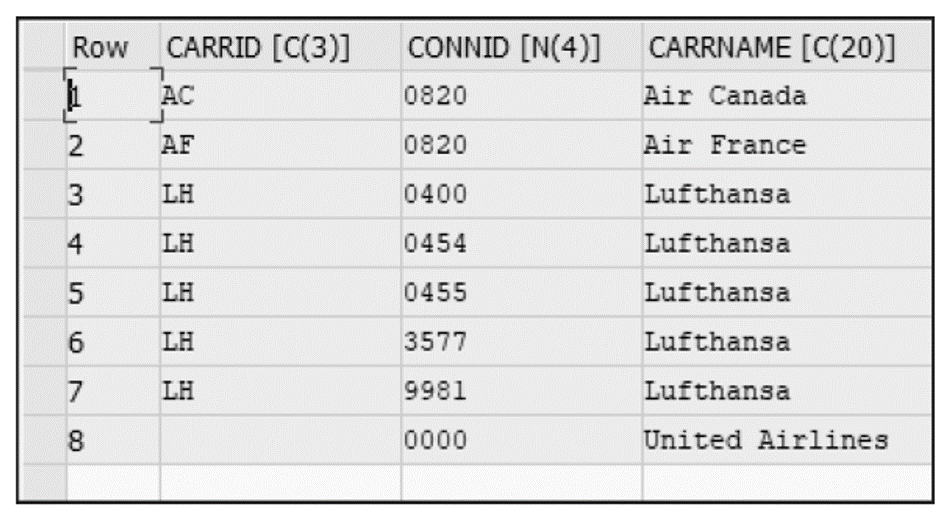### Referential Joins

A referential join is semantically an inner join that assumes that referential integrity is a given. In other words, we assume the table on the left always has a matching entry in the table on the right. Therefore, this kind of join is considered an optimized or faster inner join, since the table on the right is not checked unless a field from that table is requested. Thus, this kind of join mainly considered as a join on-demand.

In analytical views, a referential join is only executed when fields from both the tables are requested. If fields from the table on the right are selected, then the inner join is implemented. A left outer join is used if no fields from the table on the right are requested.

From a performance aspect, inner joins are slow, since the join is always executed. In contrast, with referential joins, the join is a join on-demand and only occurs if fields from the table on the right are requested. When compared with a left outer join, the performance of both joins are equally fast.

Before version SAP HANA 1.0 SP11, a referential join was only possible with a star join node. However, since version SP11, a referential join has been possible with any type of join in calculation views.

However, you should be cautious with its usage because this kind of join assumes referential integrity and can give incorrect results since the column aggregations may vary depending on the columns considered.

A referential join should only be used when you are 100% sure that, for each row in one table, there is at least one corresponding entry in the other table. Additionally, this fact must be true in both directions and at all times. All referential joins share a few characteristics:

• Reliance on referential integrity: Guarantees each entry in the table on the left has a corresponding row entry in the table on the right.
• Optimized for performance: Useful for joins on-demand since the join is only executed if at least one field from the table on the right is requested.
• Resemblance to an inner join, if the join used: When fields from both tables are requested, an inner join is performed.

### Text Joins

A text join is for joining a text table to a master table. This kind of join typically acts as a left outer join and is generally used with SAP tables where the language column SPRAS is available.

In an attribute property, you can also maintain a specific description mapping for the end user's language.

### Temporal Join

Data with transactional data containing the time column values and the master data containing the time validity columns.

### Star Join

A star join is a unique feature in which a calculation view is joined with multiple fact tables. This kind of join is used when various measures are needed from multiple fact tables.

A star join is more of a node type for a calculation view of type cube rather than a join, which is how the data is structured into a star schema. The data source/fact table for the star join can be any type of input node. The only acceptable dimensions in a star join are the dimensions of a calculation view of data category dimensions. The following types of joins can be used with star joins:

• Inner joins
• Left outer joins
• Right outer joins
• Referential joins
• Full outer joins
• Text joins

The figure below shows an example of a star join in which the tables used are for the SFLIGHT data model. SFLIGHT is projected into the Projection_1, and SBOOK, into Projection_2, and these projections are joined together in a join (JOIN1). The resultant join is star-joined to the calculation views CV_SCUSTOM_STAR and CV_SBOOK_STAR.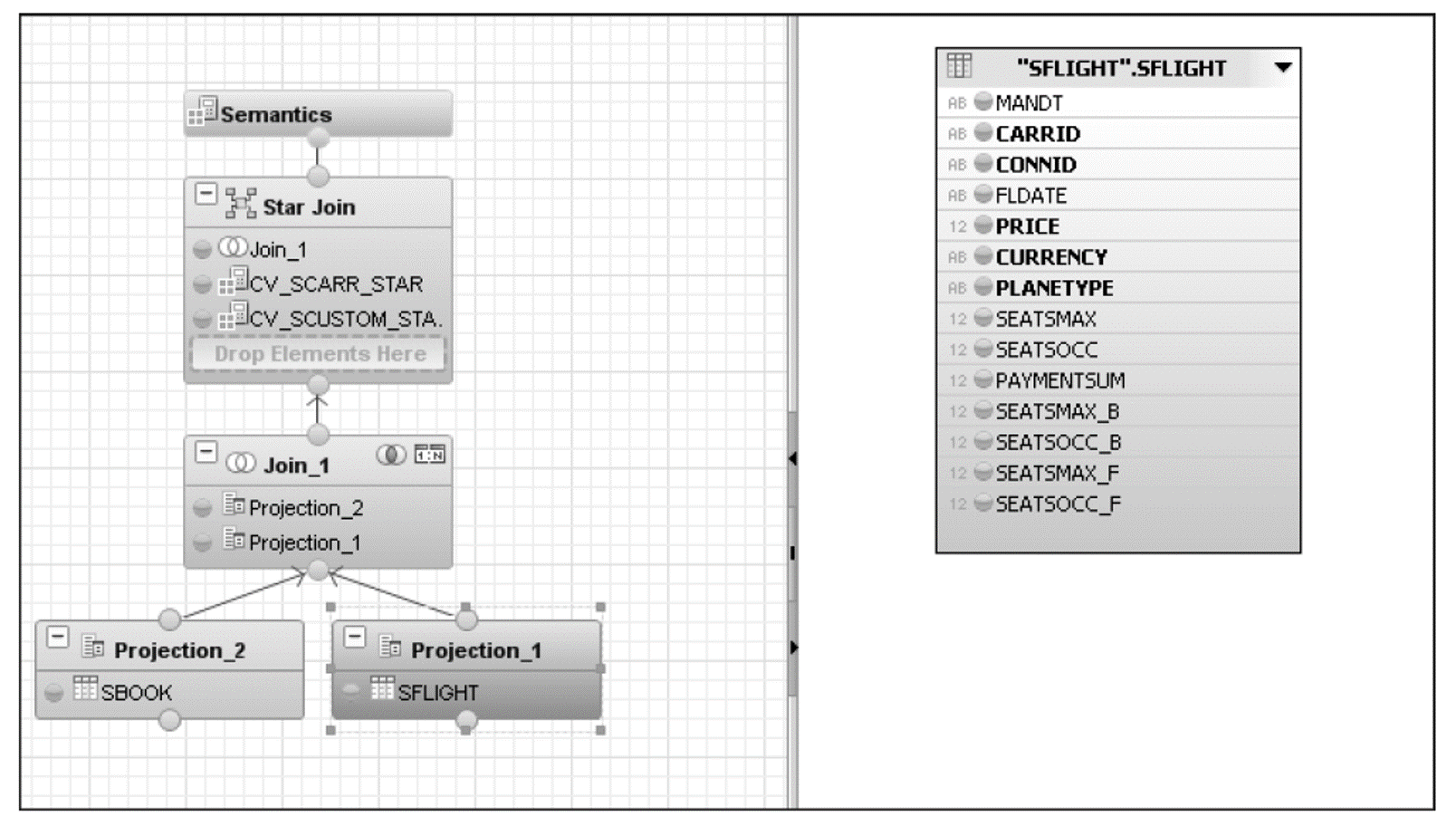The next figure shows all the fields that have been selected for each projection, and the join condition is shown in the figure following that one.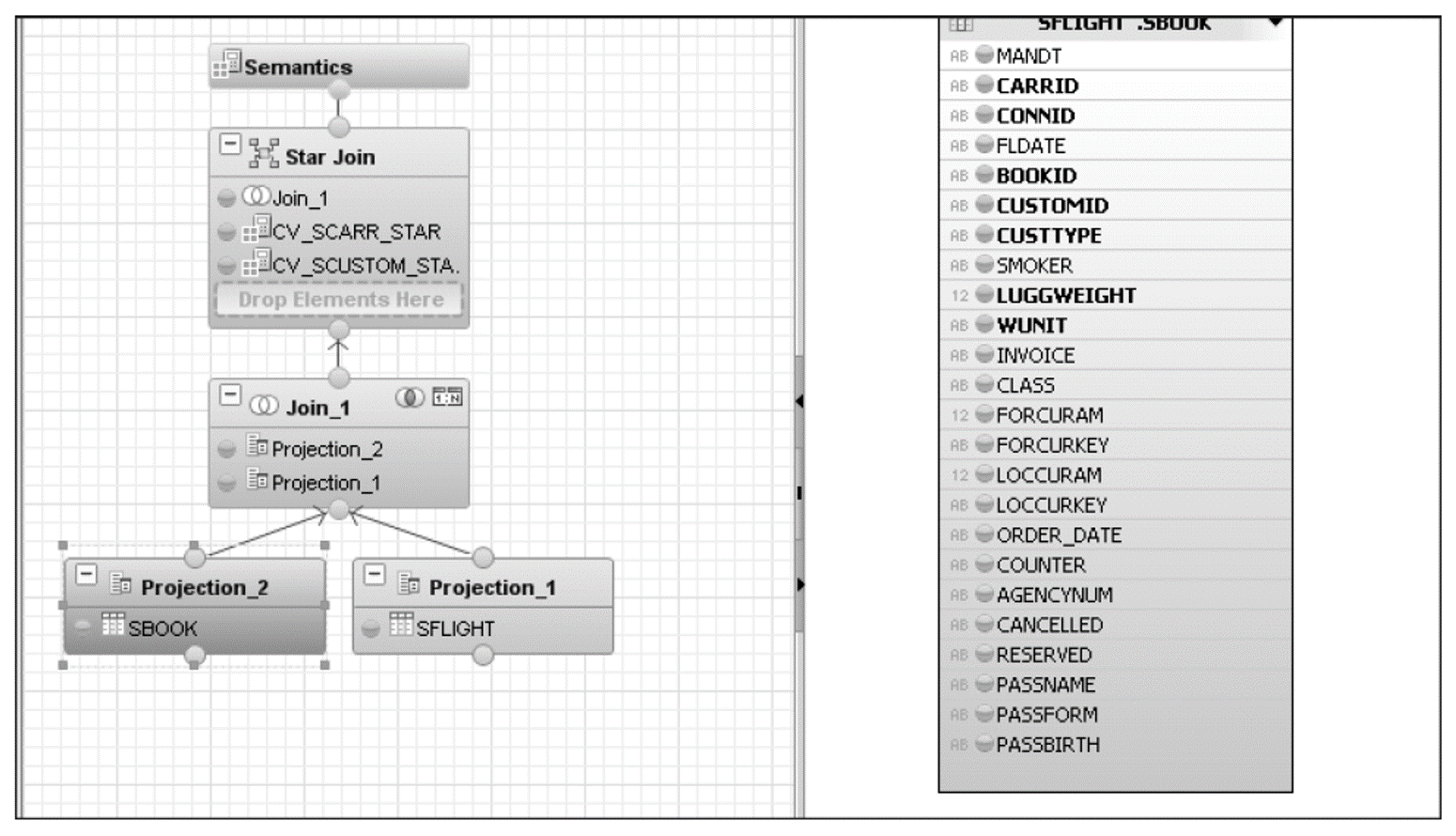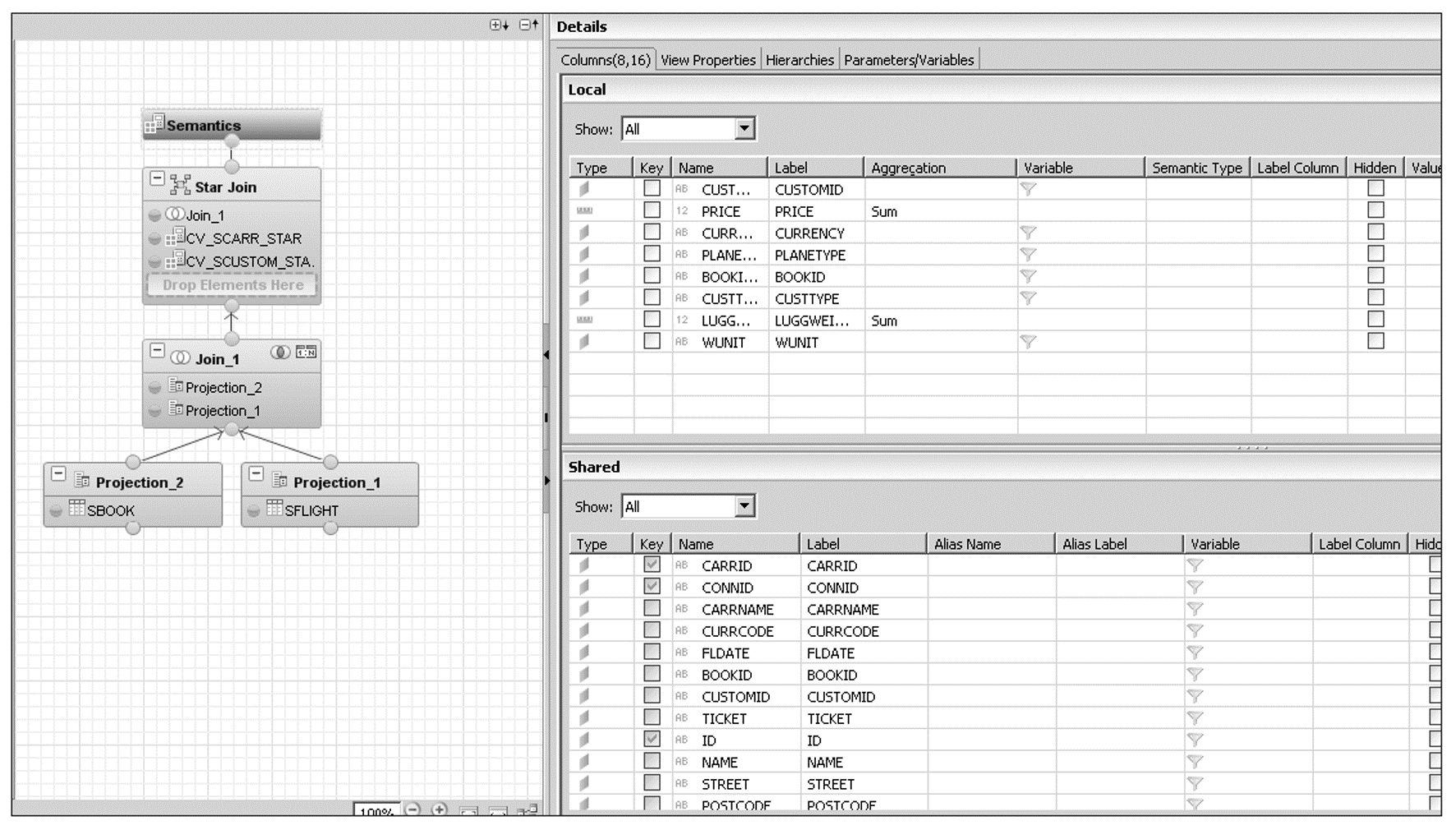Editor’s note: This post has been adapted from a section of the book ABAP Development for SAP HANA by Mohd Mohsin Ahmed and Sumit Dipak Naik.

## Recommendation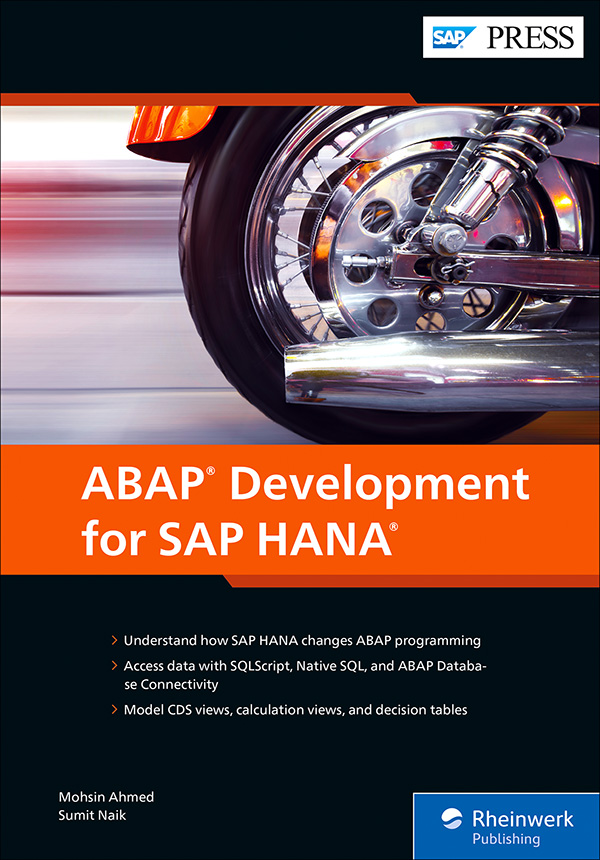ABAP Development for SAP HANA

This step-by-step developer’s guide has everything you need to build SAP HANA-optimized ABAP applications. Explore the environment and tools you’ll be using and master development techniques that govern the code-to-data paradigm in SAP HANA. Then jump into backend programming using ABAP Database Connectivity, SQLScript, ABAP-managed database procedures, core data services, and more. Once you’ve coded your apps, maintain them with runtime statistics, traces, and code analysis. Build it your way!